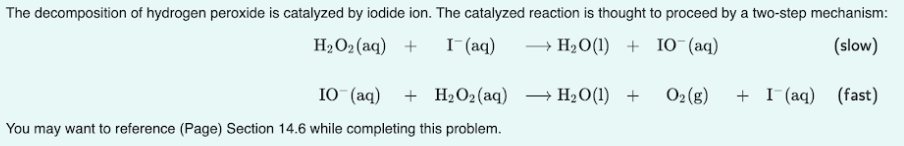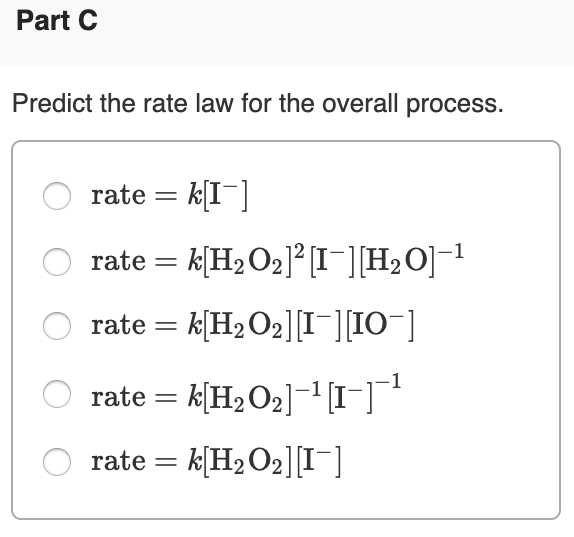# The decomposition of hydrogen peroxide is catalyzed by iodide ion. The catalyzed reaction is thought to proceed by a two-step mechanism: H2O2(aq) + I^-(aq) → H2O(l) + IO^-(aq) (slow) IO^-(aq) + H2O2(aq) → H2O(l) + O2(g) + I^-(aq) (fast) You may want to reference (page) Section 14.6 while completing this problem. Predict the rate law for the overall process. a) rate = k[I6-] b) rate = k[H2O2]^2[I^-][H2O]^-1 c) rate = k[H2O2][I^-][IO^-] d) rate = k[H2O2]^-1[I^-]^-1 e) rate = k[H2O2][I^-]